Next: Capillary Waves Up: Waves in Incompressible Fluids Previous: Steady Flow over a

# Surface Tension

As described in Chapter 3, there is a positive excess energy per unit area,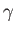, associated with an interface between two immiscible fluids. The quantitycan also be interpreted as a surface tension. Let us now incorporate surface tension into our analysis. Suppose that the interface lies at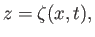(11.112)

where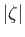is small. Thus, the unperturbed interface corresponds to the plane. The unit normal to the interface is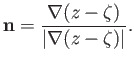(11.113)

It follows that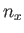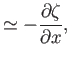(11.114)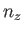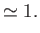(11.115)

The Young-Laplace Equation (see Section 3.2) yields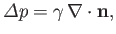(11.116)

whereis the jump in pressure seen crossing the interface in the opposite direction to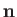. However, from Equations (11.114) and (11.115), we have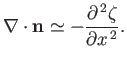(11.117)

Hence, Equation (11.116) gives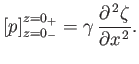(11.118)

This expression is the generalization of Equation (11.99) that takes surface tension into account.

Suppose that the interface in question is that between a body of water, of densityand depth, and the atmosphere. Let the unperturbed water lie between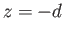and, and let the unperturbed atmosphere occupy the region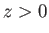. In the limit in which the density of the atmosphere is neglected, the pressure in the atmosphere takes the fixed value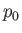, whereas the pressure just below the surface of the water is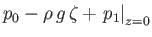. Here,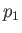is the pressure perturbation due to the wave. The relation (11.118) yields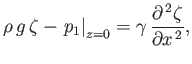(11.119)

whereis the surface tension at an air/water interface. However,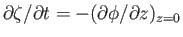, where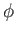is the perturbed velocity potential of the water. Moreover, from Equation (11.9),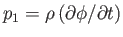. Hence, the previous expression gives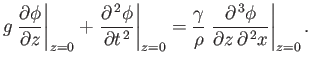(11.120)

This relation, which is a generalization of Equation (11.15), is the condition satisfied at a free surface in the presence of non-negligible surface tension. Applying this boundary condition to the general solution, (11.19) (which already satisfies the boundary condition at the bottom), we obtain the dispersion relation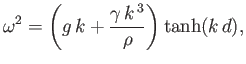(11.121)

which is a generalization of Equation (11.21) that takes surface tension into account.Next: Capillary Waves Up: Waves in Incompressible Fluids Previous: Steady Flow over a
Richard Fitzpatrick 2016-03-31